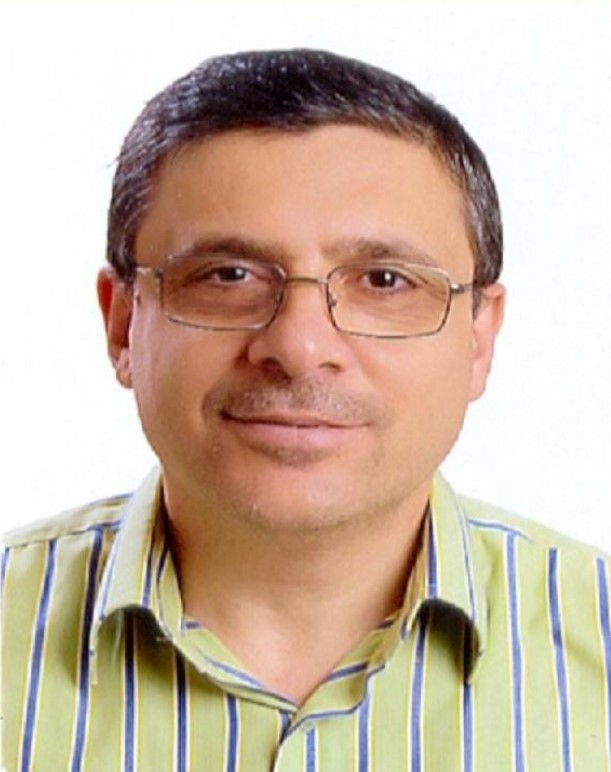Dr. Marwan Abualrub Senior Lecturer
Telephone 0555303818
Bio
Education
Teaching Areas
Research Interests
Bio

### Senior Lecturer, Preparatory Program

Dr. Marwan Abualrub has 35 years of academic experience at 7 worldwide universities.

Education
• Ph.D. in Applied Mathematics
Teaching Areas

All Mathematics and some Statistics courses...sample of courses taught:

MATH 901:Advanced Topics in Applied Mathematics for Ph.D. students,
MATH 903:Advanced Partial Differential Equations with Applications for Math and Physics Ph.D. Students,
Basic Mathematics, College Algebra, Precalculus, Trigonometry, Calculus I, Calculus II, Calculus III, Calculus I for Engineering students, Calculus II for Engineering students, Mathematics for finance, Business Calculus, Mathematics for Business, Business Statistics, Statistics 1, Statistics & Probability, Mathematical Statistics, Vector Calculus, Advanced Calculus, Business Calculus, Ordinary Differential Equations I, Differential Equations II and modeling, Differential Equations for Engineering students, Linear Algebra, Applied Mathematics for Engineering students, Advanced Engineering Mathematics I & II, Partial Differential Equations I & II, Complex Variables, Mathematics for Biology 1 & II, Real Analysis I&II, Advanced mathematical methods, Numerical Analysis, Special Course in Modeling, Graduation Research Projects in Partial Differential Equations and in Mathematical Biology (AIDS Modeling). Information Technology I, Information Technology II, Information Technology III.

Research Interests
• Applied Mathematics